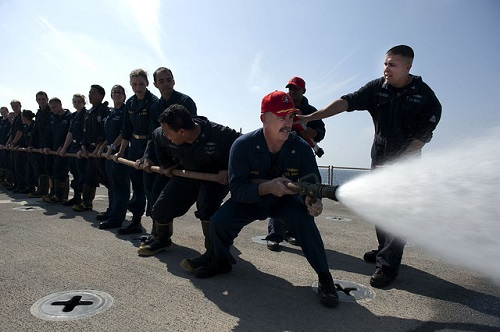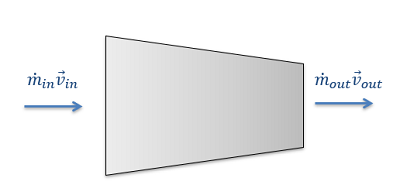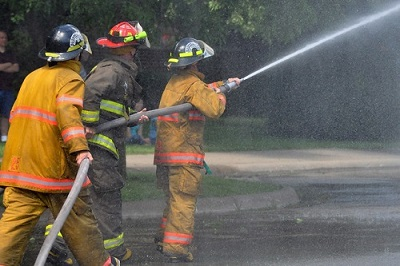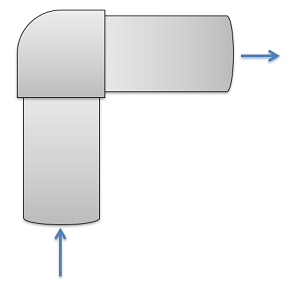﻿ Mechanics Map - Steady Flow Devices

A steady flow device is any device that will have a continuous flow of material through it. Some examples of steady flow devices include pipes, nozzles, diffusers, and pumps. Generally the material flowing through the device is a gas or liquid, and if the device in any way changes the velocity of the fluid then that fluid will exert a force on the steady flow device in return.The nozzle on the fire hose is an example of a steady flow device. Because the nozzle changes the velocity of the water as it exits the hose, it will take a force to hold the nozzle in place.

In order to determine the forces at play on a steady flow device, we will start with our impulse and momentum equation.

 $\vec{J} = m \vec{v_{f}}-m \vec{v_{i}}$

Because this is a continuous process, it doesn't really make sense to have initial and final velocities. Instead we will have an inlet and outlet velocities. Also, the mass will need to be changed to the mass flow rate (the mass entering or leaving the device per unit time) to deal with the continuous flow nature of the system.The mass flow rate is a more appropriate measure for steady flow devices than standard mass.

Dividing our initial impulse-momentum equation by time on both sides will give us the desired mass flow rate on the right, while the time on the left will cancel out the time component of the impulse.

 $\frac{\vec{F}*t}{t} = \frac{m_{out}}{t} \vec{v_{out}}-\frac{m_{in}}{t} \vec{v_{in}}$

Simplifying this equation we will arrive at our final equation, which relates the force our steady flow device exerts on the fluid to the mass flow rates and velocities at the inlet and outlet. The force the fluid exerts on the device would simply be equal and opposite to the force below.

 $\vec{F} = \dot{m}_{out} \vec{v_{out}}-\dot{m}_{in} \vec{v_{in}}$

One final note is that these equations are vector equations. If the device is changing the direction of the flow of a fluid you will need to break the force and velocities into x and y components and split the above equation into x and y components.

## Finding Mass Flow Rate:

If the mass flow rate in or out of your device is not given directly, you may need to find those values. First we can use a simple identity, knowing the mass flow rate will be equal to the density of the fluid times the volumetric flow rate. Furthermore, the volumetric flow rate can be related to the geometry of the device, in that it will be equal to the average velocity of the fluid at the inlet or outlet times the cross sectional area at the inlet or outlet. Putting this all together, we arrive at the following formulas.

 $\dot{m}= \rho \dot{V} = \rho \vec{v} A$

## Worked Problems:

### Question 1:

A fire fighter supports a hose as shown below. The hose has a volumetric flow rate of 60 gal/min and the nozzle reduces in diameter from 4 cm to 2 cm. What force will the fire fighter have to exert, in Newtons, to keep the hose in place?Photo by Macomb Paynes. License: CC BY-NC-SA 2.0

### Question 2:

A 90-degree elbow joint redirects the flow along a 3 cm diameter pipe. If water (density=1000 kg/m3) is traveling through the pipe with an average speed of 5 m/s, what is the magnitude and direction of the force the water exerts on the elbow joint?